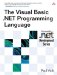# Conditional Statements

 <  Day Day Up  >

Conditional statements allow executing code depending on whether some situation is true or false. They are fundamental to the decision making processes within a program.

#### If Statement

The If statement is a simple conditional statement that executes code based on whether a condition is true or false. The expression in an If statement must to evaluate to a Boolean value ”if the expression evaluates to True , the statements contained between the If statement and an End If statement are executed. If the expression evaluates to False , the statements are not executed.

` If x > 0 Then   Console.WriteLine("x is greater than zero.") End If `

The block can also contain a block that starts with the keyword Else . If the expression evaluates to False , the Else block will be executed instead of the If block.

` If x > 0 Then   Console.WriteLine("x is greater than zero.") Else   Console.WriteLine("x is less than or equal to zero.") End If `

It is also possible to chain If statements together by using the ElseIf keyword. The If statements will be evaluated in order from first to last. The first If statement to evaluate to True will be executed (or the Else block will be executed if there is one and no If statement evaluates to True ).

` If x > 0 Then   Console.WriteLine("x is greater than zero.") ElseIf x < 0 Then   Console.WriteLine("x is less than zero.") Else   Console.WriteLine("x is zero.") End If `

An If statement can also be written entirely on one line ”in that case, no End If is necessary. It is not possible to use an ElseIf in a line If statement, but Else can be used.

` If x > 0 Then y = x If y < 0 Then z = y Else z = 5 `

Note that all the statements that follow the Then or Else in a line if are considered part of the If statement. In the following example, nothing will be printed.

` x = 0 If x > 0 Then x = x + 1 : Console.WriteLine("x is greater than zero.") `

#### Select Statement

Often a decision needs to be made not on the basis of a simple true/false condition but by choosing between a set of different possibilities. The Select statement simplifies that process. A Select statement begins with the keywords Select Case and an expression that forms the basis of the following comparisons. The statement contains a set of Case statements that are tests for the Select expression. In its simplest form, a Case statement is just the Case keyword followed by an expression to compare the Select expression against. If the values are equal, the code between the Case statement and the next Case statement is evaluated. A Select statement is ended by an End Select statement.

` Select Case x   Case 0     Console.WriteLine("x is zero")   Case 1     Console.WriteLine("x is one")   Case 2     Console.WriteLine("x is two")   Case 3     Console.WriteLine("x is three") End Select `

Any value that can be compared using the = operator can be used in a Select statement.

` Select Case x   Case "Red"     Console.WriteLine("x is red")   Case "Green"     Console.WriteLine("x is green")   Case "Blue"     Console.WriteLine("x is blue") End Select `

The Case statements are compared in order from first to last ”once a Case statement evaluates to True , no further comparisons are done. In the following example, the third case statement will never be executed.

` Select Case x   Case 0     Console.WriteLine("x is zero")   Case 1     Console.WriteLine("x is one")   Case 1     Console.WriteLine("x is one, also") End Select `

Ranges of numeric values can be compared by specifying a value, followed by the To keyword and another value. If the Select expression is within that range, the Case statement will be executed.

` Select Case x   Case 0     Console.WriteLine("x is zero")   Case 1 To 5     Console.WriteLine("x is one, two, three, four or five")   Case 6 To 10     Console.WriteLine("x is six, seven, eight, nine or ten") End Select `

If a comparison other than equality is needed, the Case keyword can be followed by the Is keyword, a comparison operator, and an expression. This causes the specified comparison to be performed. Also, a Case Else statement will be executed if none of the other Case statement evaluates to True . For example:

` Select Case x   Case Is < 0     Console.WriteLine("x is less than zero")   Case 0     Console.WriteLine("x is zero")   Case Else     Console.WriteLine("x is greater than zero") End Select `

Finally, multiple cases can be combined by separating the Case expressions with commas. For example:

` Select Case x   Case 1, 3, 5, 7     Console.WriteLine("x is prime")   Case Is < 0, Is > 10     Console.WriteLine("x is out of range")   Case Else     Console.WriteLine("x is not prime") End Select `
 <  Day Day Up  >The Visual Basic .NET Programming Language
ISBN: 0321169514
EAN: 2147483647
Year: 2004
Pages: 173
Authors: Paul Vick

Similar book on Amazon